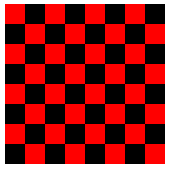### Programming Exercises For Chapter 3

THIS PAGE CONTAINS programming exercises based on material from Chapter 3 of this on-line Java textbook. Each exercise has a link to a discussion of one possible solution of that exercise.

Exercise 3.1: How many times do you have to roll a pair of dice before they come up snake eyes? You could do the experiment by rolling the dice by hand. Write a computer program that simulates the experiment. The program should report the number of rolls that it makes before the dice come up snake eyes. (Note: "Snake eyes" means that both dice show a value of 1.) Exercise 2.2 explained how to simulate rolling a pair of dice.

See the solution!

Exercise 3.2: Which integer between 1 and 10000 has the largest number of divisors, and how many divisors does it have? Write a program to find the answers and print out the results. It is possible that several integers in this range have the same, maximum number of divisors. Your program only has to print out one of them. One of the examples from Section 3.4 discussed divisors. The source code for that example is CountDivisors.java.

You might need some hints about how to find a maximum value. The basic idea is to go through all the integers, keeping track of the largest number of divisors that you've seen so far. Also, keep track of the integer that had that number of divisors.

See the solution!

Exercise 3.3: Write a program that will evaluate simple expressions such as 17 + 3 and 3.14159 * 4.7. The expressions are to be typed in by the user. The input always consist of a number, followed by an operator, followed by another number. The operators that are allowed are +, -, *, and /. You can read the numbers with TextIO.getDouble() and the operator with TextIO.getChar(). Your program should read an expression, print its value, read another expression, print its value, and so on. The program should end when the user enters 0 as the first number on the line.

See the solution!

Exercise 3.4: Write a program that reads one line of input text and breaks it up into words. The words should be output one per line. A word is defined to be a sequence of letters. Any characters in the input that are not letters should be discarded. For example, if the user inputs the line

```          He said, "That's not a good idea."
```

then the output of the program should be

```          He
said
that
s
not
a
good
idea
```

(An improved version of the program would list "that's" as a word. An apostrophe can be considered to be part of a word if there is a letter on each side of the apostrophe. But that's not part of the assignment.)

To test whether a character is a letter, you might use (ch >= 'a' && ch <= 'z') || (ch >= 'A' && ch <= 'Z'). However, this only works in English and similar languages. A better choice is to call the standard function Character.isLetter(ch), which returns a boolean value of true if ch is a letter and false if it is not. This works for any Unicode character. For example, it counts an accented e, é, as a letter.

See the solution!

Exercise 3.5: Write an applet that draws a checkerboard. Assume that the size of the applet is 160 by 160 pixels. Each square in the checkerboard is 20 by 20 pixels. The checkerboard contains 8 rows of squares and 8 columns. The squares are red and black. Here is a tricky way to determine whether a given square is red or black: If the row number and the column number are either both even or both odd, then the square is red. Otherwise, it is black. Note that a square is just a rectangle in which the height is equal to the width, so you can use the subroutine g.fillRect() to draw the squares. Here is an image of the checkerboard:(To run an applet, you need a Web page to display it. A very simple page will do. Assume that your applet class is called Checkerboard, so that when you compile it you get a class file named Checkerboard.class Make a file that contains only the lines:

```           <applet code="Checkerboard.class" width=160 height=160>
</applet>
```

Call this file Checkerboard.html. This is the source code for a simple Web page that shows nothing but your applet. You can open the file in a Web browser or with Sun's appletviewer program. The compiled class file, Checkerboard.class, must be in the same directory with the Web-page file, Checkerboard.html.)

See the solution!

Exercise 3.6: Write an animation applet that shows a checkerboard pattern in which the even numbered rows slide to the left while the odd numbered rows slide to the right. You can assume that the applet is 160 by 160 pixels. Each row should be offset from its usual position by the amount getFrameNumber() % 40. Hints: Anything you draw outside the boundaries of the applet will be invisible, so you can draw more than 8 squares in a row. You can use negative values of x in g.fillRect(x,y,w,h). Here is a working solution to this exercise:

Your applet will extend the non-standard class, SimpleAnimationApplet2, which was introduced in Section 7. When you run your applet, the compiled class files, SimpleAnimationApplet2.class and SimpleAnimationApplet2\$1.class, must be in the same directory as your Web-page source file and the compiled class file for your own class. These files are produced when you compile SimpleAnimationApplet2.java. Assuming that the name of your class is SlidingCheckerboard, then the source file for the Web page should contain the lines:

```           <applet code="SlidingCheckerboard.class" width=160 height=160>
</applet>

```

See the solution!

[ Chapter Index | Main Index ]